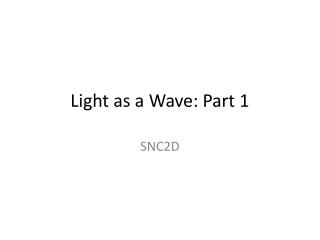# Light as a Wave: Part 1 - PowerPoint PPT PresentationDownload PresentationLight as a Wave: Part 1

Light as a Wave: Part 1Download Presentation## Light as a Wave: Part 1

- - - - - - - - - - - - - - - - - - - - - - - - - - - E N D - - - - - - - - - - - - - - - - - - - - - - - - - - -
##### Presentation Transcript

1. What is a wave? A wave is a disturbance which carries energy from one location to another. The material the disturbance travels through is the medium. The movement of the disturbance is referred to as propagation.

2. 2 Types of Waves A longitudinal wave is a wave in which the particles of the medium move in a direction parallel to the direction of propagation.

3. 2 Types of Waves A longitudinal wave is a wave in which the particles of the medium move in a direction parallel to the direction of propagation.

4. 2 Types of Waves A transverse wave is a wave in which the particles of the medium move in a direction perpendicular to the direction of propagation.

5. Snapshot of a Wave The dashed line represents the rest positions of the particles.

6. Snapshot of a Wave The positions of maximum displacement are referred to as crests (positive displacement) and troughs (negative). The maximum displacement is the amplitude.

7. Snapshot of a Wave The distance between one crest and the next crest (or one trough and the next trough) is the

8. Snapshot of a Wave The distance between one crest and the next crest (or one trough and the next trough) is the wavelength, represented by

9. Snapshot of a Wave The distance between one crest and the next crest (or one trough and the next trough) is the wavelength, represented by l.

10. Snapshot of a Wave The time it takes one complete wavelength to pass a single point is the

11. Snapshot of a Wave The time it takes one complete wavelength to pass a single point is the period, represented by

12. Snapshot of a Wave The time it takes one complete wavelength to pass a single point is the period, represented by T.

13. Snapshot of a Wave The number of complete wavelengths that pass a single point in one second is the frequency, represented by f.

14. Frequency Frequency is measured in units of s-1 or Hertz (Hz). “I do not think that the wireless waves I have discovered will have any practical application.”

15. Wave Speed The speed of a wave is therefore:

16. Wave Speed The speed of a wave is therefore:

17. Wave Speed The speed of a wave is therefore:

18. Wave Speed The speed of a wave is therefore: Practice Question: What is the wavelength of a wave with a speed of 344 m/s and a frequency of 256 Hz?

19. Wave Speed Practice Question: What is the wavelength of a wave with a speed of 344 m/s and a frequency of 256 Hz?

20. Wave Speed Practice Question: What is the wavelength of a wave with a speed of 344 m/s and a frequency of 256 Hz?

21. Wave Speed Practice Question: What is the wavelength of a wave with a speed of 344 m/s and a frequency of 256 Hz?

22. Wave Speed Practice Question: What is the wavelength of a wave with a speed of 344 m/s and a frequency of 256 Hz?

23. Wave Speed Practice Question: What is the wavelength of a wave with a speed of 344 m/s and a frequency of 256 Hz?

24. Wave Speed Practice Question: What is the wavelength of a wave with a speed of 344 m/s and a frequency of 256 Hz?

25. Light is an “EM” wave Light is actually a transverse wave

26. Light is an “EM” wave Light is actually a transverse wave, but the perpendicular-to-propagation disturbance is not of particles of a medium but of the electric and magnetic (EM) fields

27. The Speed of Light Because light waves do not need a medium, they can travel through a vacuum (empty space) and do so at the speed of light: c =

28. The Speed of Light Because light waves do not need a medium, they can travel through a vacuum (empty space) and do so at the speed of light: c = 3.0 x 108 m/s.

29. Light-years A light-year is the distance light would travel in 1 year.

30. Light-years A light-year is the distance light would travel in 1 year. Practice Question: How far (in m) is 1.0 light-years?

31. Light-years A light-year is the distance light would travel in 1 year. Practice Question: How far (in m) is 1.0 light-years?

32. The Speed(s) of Light Light will travel slower in denser media (e.g. glass), but the speed in air is still effectively 3.0 x 108 m/s and

33. More Practice p. 391 #1 and 2